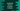# JavaScript program to check if a number is Moran## JavaScript program to check if a number is Moran or not:

In this post, we will write a JavaScript program to check if a number is a Moran number or not. We will learn what is a Moran number and how to write a program in JavaScript to check for Moran numbers.

The program will take one number as input from the user to check if it is Moran or not.

### Moran number:

A number is called a Moran number if the result of dividing the number by its sum is prime. For example, 45 is a Moran number. Because,

• The sum of digits of this number is 9.
• If we divide 45 by 9, the result is 5, which is a prime number.

Similarly, 46 is not a Moran number because the division result of 46/10 is not prime.

Let’s write down the program now.

### JavaScript program to check for Moran number:

The following JavaScript program can be used to check if a number is a Moran number or not:

``````function isPrime(n) {
for (let i = 2; i <= n / 2; i++) {
if (n % i == 0) return false;
}
return true;
}

function findDigitsSum(n) {
let sum = 0;
while (n) {
sum += n % 10;
n = Math.floor(n / 10);
}
return sum;
}

function isMoran(n) {
let sumDigits = findDigitsSum(n);
if (n % sumDigits !== 0) return false;

return isPrime(n / sumDigits);
}

let no = 45;

if (isMoran(no)) {
console.log(`\${no} is a Moran number.`);
} else {
console.log(`\${no} is not a Moran number.`);
}``````

Here,

• The isPrime function is used to check if a number is prime or not. It uses a for loop to check if a number is prime. It returns one boolean value.
• The function findDigitsSum is used to find the sum of digits of a number. It uses a while loop to find the sum of digits of the number.
• The isMoran function checks if a number is a Moran number or not. It uses the findDigitsSum function to find the sum of digits of the number and assigns that value to the sumDigits variable. If n is not perfectly divisible by sumDigits, it returns false. Else, it checks if the value of n/sumDigits is prime or not. It is a Moran number if the value is prime, else not.

This program is checking if 45 is Moran or not.

If you run this program, it will print:

``45 is a Moran number.``